Computer Science

# Practice with while loopsThe black squares represent the ground. The green squares represent the branches of a tree. The red square represents the trunk of a tree — except it is not complete!

Your job is to fix the tree so that it looks like this:## Starter code

Download the zip file above and put it in your `bit` folder. You will find a file called `fix_tree.py` that has the following starter code:

``````from byubit import Bit

@Bit.worlds('fix-tree', 'fix-another-tree')
def fix_the_tree(bit):
# Write code here
pass

if __name__ == '__main__':
fix_the_tree(Bit.new_bit)``````

## Planning

Before you write any code, you should plan what you want to do. A good way to do this is with pencil and paper. Find a friend and draw out how you think you would solve this problem.What did you draw? Maybe you drew something like this: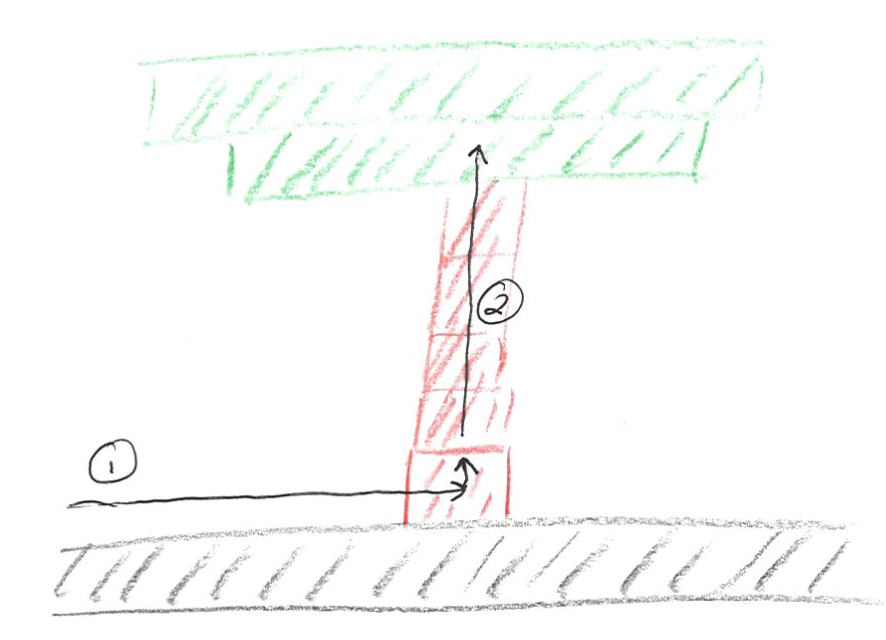• Step 1: move to the tree trunk and turn left
• Step 2: draw the rest of the tree trunk

## Decomposing the problem

Remember from [/guide/unit1/practice-with-functions](Practice with functions), it is good practice to start at the highest or most abstract level and write functions. For example:

``````def fix_the_tree(bit):
""" Move to the trunk and the draw the trunk. """
move_to_the_trunk(bit)
draw_the_trunk(bit)``````

Remember, PyCharm will put squiggly red lines underneath the functions you haven’t defined yet: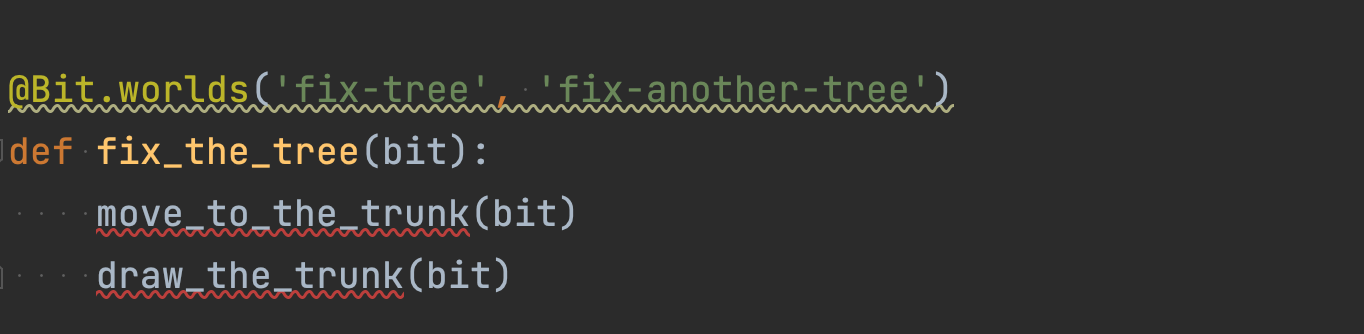Hover over these and select `Create function` in blue to create empty functions for each of them:

``````def move_to_the_trunk(bit):
pass

def draw_the_trunk(bit):
pass

@Bit.worlds('fix-tree', 'fix-another-tree')
def fix_the_tree(bit):
""" Move to the trunk and the draw the trunk. """
move_to_the_trunk(bit)
draw_the_trunk(bit)``````

## Moving to the trunk

How would you move to the trunk? Hint: remember what we learned in the guide on while. Also, remember you want to turn left after you get to the trunk, so you can be in position to draw the trunk in the next step.Here is one way to do this:

``````def move_to_the_trunk(bit):
""" Move until Bit gets to a red square, then turn left. """
while not bit.is_red():
bit.move()

bit.left()``````

Let’s stop here, run the code, and see how we are doing: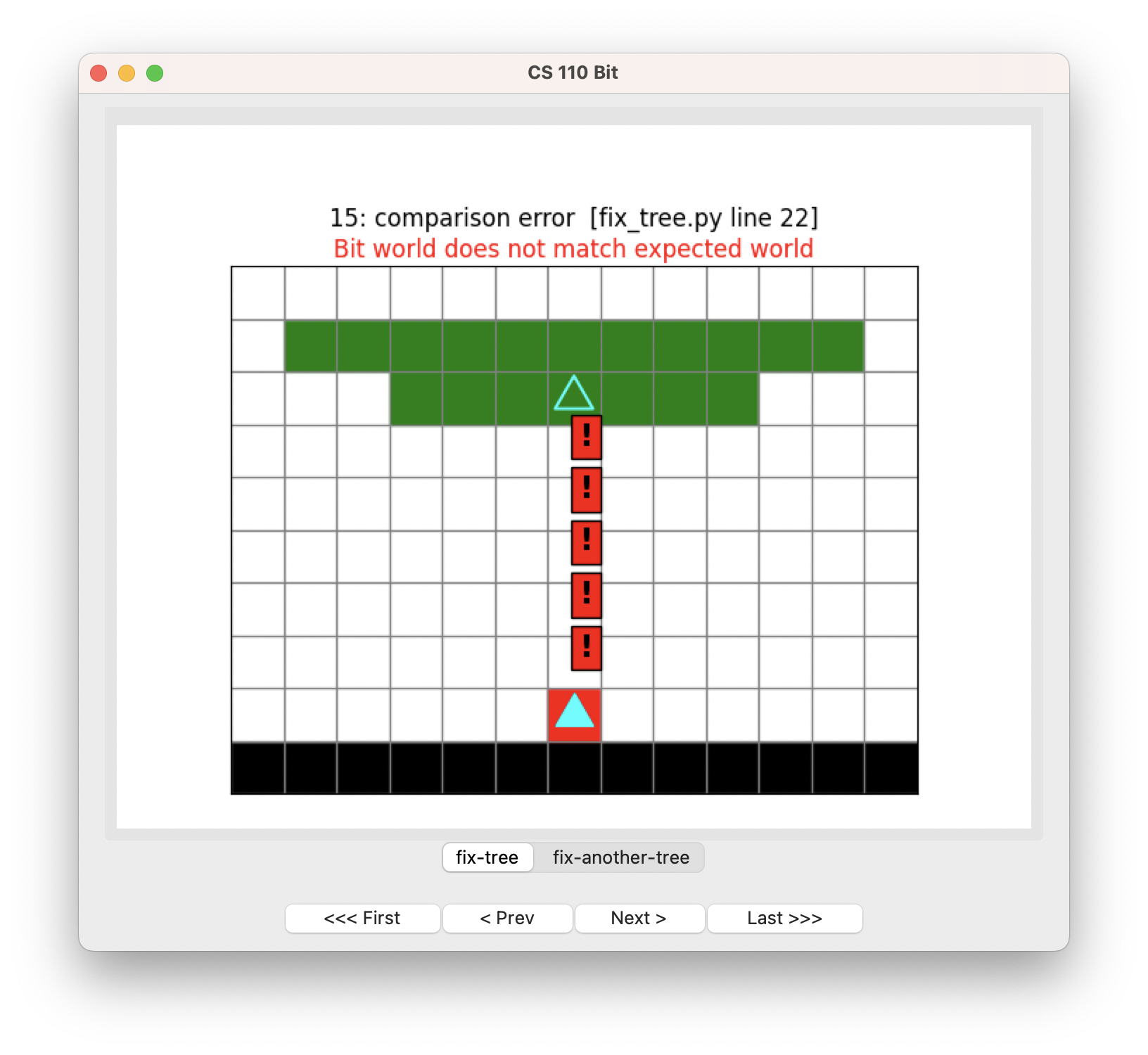Fantastic!

Work on problems one step at a time. Don’t try to solve the whole problem at once.

Remember, we can do this if we define our functions first, and then put `pass` in the ones we don’t want to implement yet.

## Drawing the trunk

How would you move to the trunk?Here is one way to try to do this:

``````def draw_the_trunk(bit):
""" Move until Bit gets to a green square, painting red on the way. """
while not bit.is_green():
bit.move()
bit.paint('red')``````

This code makes sense — as long as we are not on a green square, move and paint red for the trunk.

But here is what happens when we run this:Oops! We somehow painted right through the trunk and tried to go out of bounds!

If this was your first try, don’t be embarrassed. Everybody makes mistakes like this all the time. The key is understanding why you made a mistake and being able to fix it. To a large extent, making and fixing mistakes is what coding is all about. (It’s also a lot about what life is like.)

Use the `First` and `Next` buttons. See if you can figure out why this code doesn’t work.If you get to this step: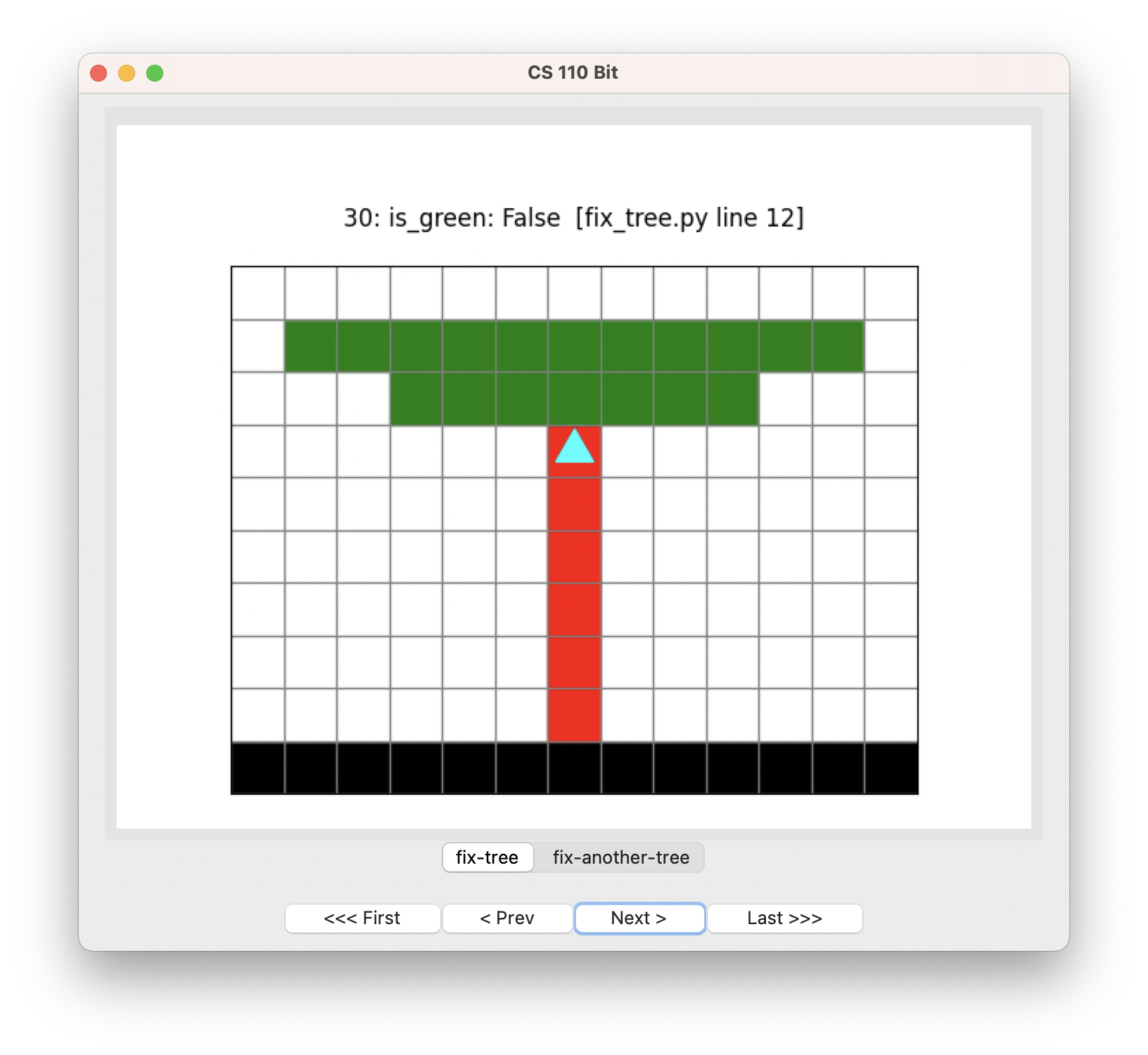click `Next` slowly and observe each step. You will observe that Python runs your code this way:

• is the current square green? no
• move
• paint red
• is the current square green? no because we just painted it red

Aha! So we need to rethink our code. The loop can’t move and then paint red, because it will always change the tree branches to be red.

So let’s try reversing the order of those statements:

``````def draw_the_trunk(bit):
""" Move until Bit gets to a green square, painting red on the way. """
while not bit.is_green():
bit.paint('red')
bit.move()``````

Run this code: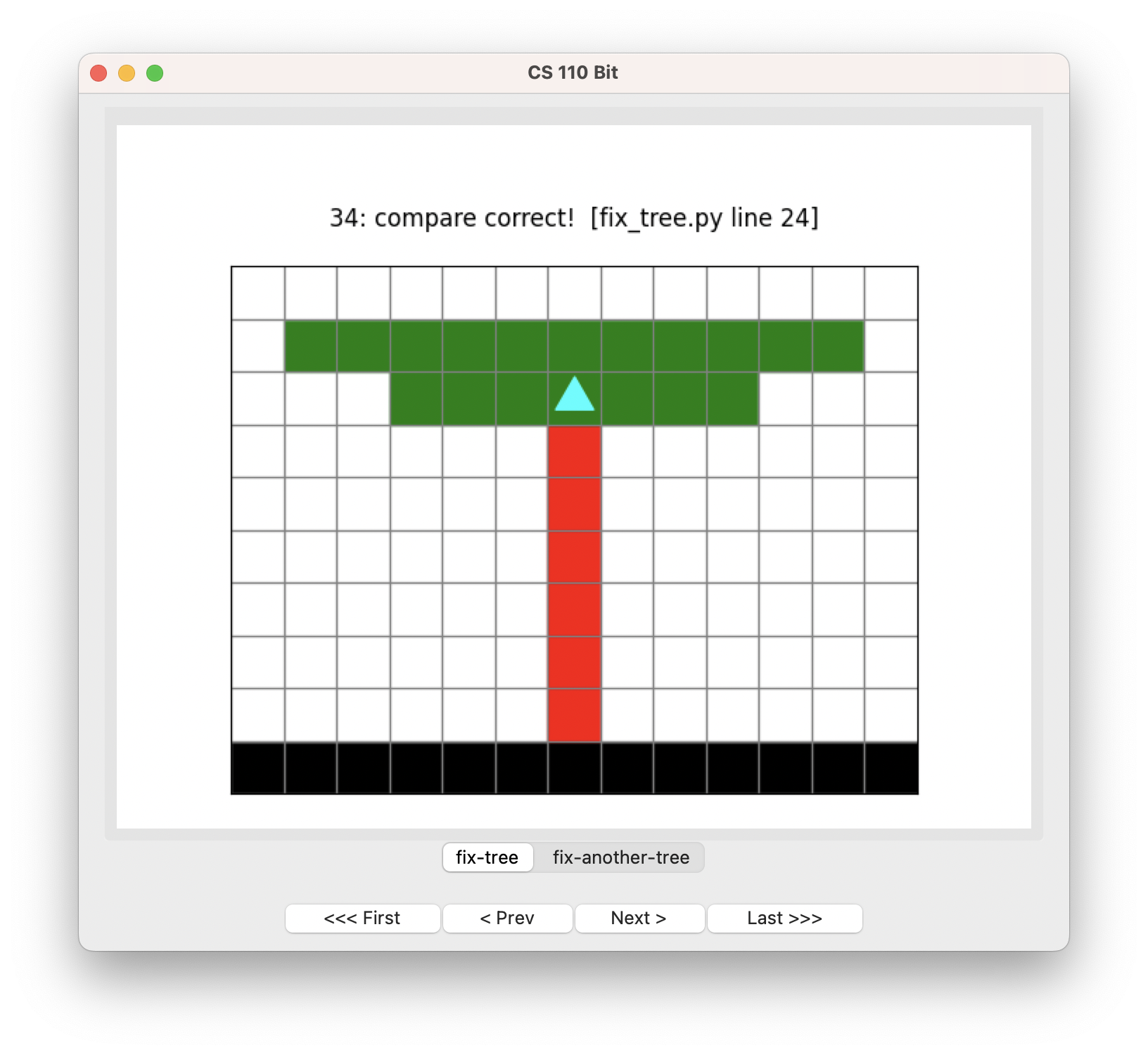Hooray!

Be sure to click on the `fix-another-tree` tab and be sure your solution works for the other world:## Getting picky

You may have noticed something unusual about our solution:

``````def draw_the_trunk(bit):
""" Move until Bit gets to a green square, painting red on the way. """
while not bit.is_green():
bit.paint('red')
bit.move()``````

When we run this function, Bit is already on a red square, and then we paint it red again. That’s not the end of the world, obviously. It is OK to repaint a square as long as it stays the same color. But if you want to be picky about your code (which is a good quality to have), you could solve the problem this way:

``````def draw_the_trunk(bit):
""" Move until Bit gets to a green square, painting red on the way. """
# move one square first because we are already on red
bit.move()
while not bit.is_green():
bit.paint('red')
bit.move()``````